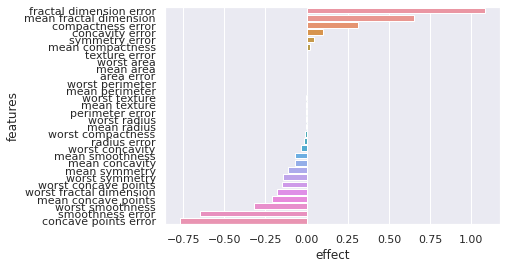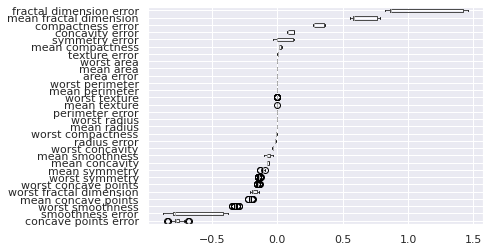Contents

# 0 - Import packages

!pip install querier # A query language for Python Data Frames (part of Techtonique)

!pip install mlsauce # Miscellaneous Statistical/Machine Learning stuff (part of Techtonique)

!pip install GPopt # Bayesian optimization using Gaussian Process Regression (part of Techtonique)

!pip install the-teller # Model-agnostic Statistical/Machine Learning explainability (part of Techtonique)

! pip install scikit-learn

!pip install SQLAlchemy

!pip install matplotlib==3.1.3 # this version is required

import numpy as np
import matplotlib.pyplot as plt
import sqlite3
import pandas as pd
import sqlalchemy
import matplotlib.pyplot as plt
import matplotlib.style as style
from sklearn.model_selection import train_test_split, cross_val_score, RepeatedKFold
from sklearn.metrics import classification_report, confusion_matrix
from time import time

import querier as qr
import GPopt as gp
import mlsauce as ms
import teller as tr


# 1 - Data-wrangling (using the querier)

Remark: Some querier verbs were tested on macOS and Linux so far (experimental).

breast_cancer = load_breast_cancer(as_frame=True)

print(breast_cancer.DESCR)

.. _breast_cancer_dataset:

Breast cancer wisconsin (diagnostic) dataset
--------------------------------------------

**Data Set Characteristics:**

:Number of Instances: 569

:Number of Attributes: 30 numeric, predictive attributes and the class

:Attribute Information:
- radius (mean of distances from center to points on the perimeter)
- texture (standard deviation of gray-scale values)
- perimeter
- area
- smoothness (local variation in radius lengths)
- compactness (perimeter^2 / area - 1.0)
- concavity (severity of concave portions of the contour)
- concave points (number of concave portions of the contour)
- symmetry
- fractal dimension ("coastline approximation" - 1)

The mean, standard error, and "worst" or largest (mean of the three
worst/largest values) of these features were computed for each image,
resulting in 30 features.  For instance, field 0 is Mean Radius, field

- class:
- WDBC-Malignant
- WDBC-Benign

:Summary Statistics:

===================================== ====== ======
Min    Max
===================================== ====== ======
texture (mean):                       9.71   39.28
perimeter (mean):                     43.79  188.5
area (mean):                          143.5  2501.0
smoothness (mean):                    0.053  0.163
compactness (mean):                   0.019  0.345
concavity (mean):                     0.0    0.427
concave points (mean):                0.0    0.201
symmetry (mean):                      0.106  0.304
fractal dimension (mean):             0.05   0.097
texture (standard error):             0.36   4.885
perimeter (standard error):           0.757  21.98
area (standard error):                6.802  542.2
smoothness (standard error):          0.002  0.031
compactness (standard error):         0.002  0.135
concavity (standard error):           0.0    0.396
concave points (standard error):      0.0    0.053
symmetry (standard error):            0.008  0.079
fractal dimension (standard error):   0.001  0.03
texture (worst):                      12.02  49.54
perimeter (worst):                    50.41  251.2
area (worst):                         185.2  4254.0
smoothness (worst):                   0.071  0.223
compactness (worst):                  0.027  1.058
concavity (worst):                    0.0    1.252
concave points (worst):               0.0    0.291
symmetry (worst):                     0.156  0.664
fractal dimension (worst):            0.055  0.208
===================================== ====== ======

:Missing Attribute Values: None

:Class Distribution: 212 - Malignant, 357 - Benign

:Creator:  Dr. William H. Wolberg, W. Nick Street, Olvi L. Mangasarian

:Donor: Nick Street

:Date: November, 1995

This is a copy of UCI ML Breast Cancer Wisconsin (Diagnostic) datasets.
https://goo.gl/U2Uwz2

Features are computed from a digitized image of a fine needle
aspirate (FNA) of a breast mass.  They describe
characteristics of the cell nuclei present in the image.

Separating plane described above was obtained using
Multisurface Method-Tree (MSM-T) [K. P. Bennett, "Decision Tree
Construction Via Linear Programming." Proceedings of the 4th
Midwest Artificial Intelligence and Cognitive Science Society,
pp. 97-101, 1992], a classification method which uses linear
programming to construct a decision tree.  Relevant features
were selected using an exhaustive search in the space of 1-4
features and 1-3 separating planes.

The actual linear program used to obtain the separating plane
in the 3-dimensional space is that described in:
[K. P. Bennett and O. L. Mangasarian: "Robust Linear
Programming Discrimination of Two Linearly Inseparable Sets",
Optimization Methods and Software 1, 1992, 23-34].

This database is also available through the UW CS ftp server:

ftp ftp.cs.wisc.edu
cd math-prog/cpo-dataset/machine-learn/WDBC/

.. topic:: References

- W.N. Street, W.H. Wolberg and O.L. Mangasarian. Nuclear feature extraction
for breast tumor diagnosis. IS&T/SPIE 1993 International Symposium on
Electronic Imaging: Science and Technology, volume 1905, pages 861-870,
San Jose, CA, 1993.
- O.L. Mangasarian, W.N. Street and W.H. Wolberg. Breast cancer diagnosis and
prognosis via linear programming. Operations Research, 43(4), pages 570-577,
July-August 1995.
- W.H. Wolberg, W.N. Street, and O.L. Mangasarian. Machine learning techniques
to diagnose breast cancer from fine-needle aspirates. Cancer Letters 77 (1994)
163-171.


Create a data frame breast_cancer_df with columns that can be used by the querier:

breast_cancer_df = breast_cancer.frame

breast_cancer_df_columns = breast_cancer_df.columns

breast_cancer_df.columns = ["_".join(elt.split()) for elt in breast_cancer_df_columns]


Querying the data frame with the querier:

Selecting

qr.select(breast_cancer_df, "mean_radius, mean_texture, mean_perimeter, mean_area, target",
limit=4, random=True)


Filtering

qr.filtr(breast_cancer_df, "(target == 1) & (mean_radius >= 10)")


Summarizing

breast_cancer_df['target'] = breast_cancer_df['target'].astype(object)

qrobj = qr.Querier(df=breast_cancer_df)

mean_concave_points,\
target")\
avg(mean_concave_points),\
target",
group_by = "target")
print(request_1.get_df())

   avg_mean_radius  avg_mean_concave_points  target
0        17.462830                 0.087990       0
1        12.146524                 0.025717       1


# 2 - Modeling/Hyperparameter tuning (using mlsauce and GPopt)

X = breast_cancer.data
y = breast_cancer.target
# split data into training test and test set
X_train, X_test, y_train, y_test = train_test_split(X, y, stratify=y,
test_size=0.2, random_state=123)


Chosen model is LSBoost. Hyperparameters tuning:

def lsboost_cv(X_train, y_train,
n_estimators=100,
learning_rate=0.1,
n_hidden_features=5,
reg_lambda=0.1,
row_sample=0.9,
col_sample=0.9,
dropout=0,
tolerance=1e-4,
seed=123):

estimator = ms.LSBoostClassifier(n_estimators=n_estimators,
activation="relu",
learning_rate=learning_rate,
n_hidden_features=n_hidden_features,
reg_lambda=reg_lambda,
row_sample=row_sample,
col_sample=col_sample,
dropout=dropout,
tolerance=tolerance,
seed=seed, verbose=0)

cv = RepeatedKFold(n_splits=5, n_repeats=3, random_state=123)

return -cross_val_score(estimator, X_train, y_train,
scoring='accuracy', cv=cv, n_jobs=4).mean()

def optimize_lsboost(X_train, y_train):

def crossval_objective(x):

return lsboost_cv(
X_train=X_train,
y_train=y_train,
n_estimators=int(x),
learning_rate=10**x,
n_hidden_features=int(x),
reg_lambda=10**x,
col_sample=x,
row_sample=x,
dropout=x,
tolerance=10**x)

gp_opt = gp.GPOpt(objective_func=crossval_objective,
lower_bound = np.array([  50, -6,   2, -2, 0.5, 0.5,   0, -6]),
upper_bound = np.array([1000, -1, 250,  5,   1,   1, 0.7, -1]),
n_init=10, n_iter=90, seed=123)

return {'parameters': gp_opt.optimize(verbose=2), 'opt_object':  gp_opt}


res_optimize_lsboost = optimize_lsboost(X_train, y_train)

best_parameters = res_optimize_lsboost['parameters']

start = time()

estimator_breast_cancer = ms.LSBoostClassifier(n_estimators=int(best_parameters),
learning_rate=10**best_parameters,
n_hidden_features=int(best_parameters),
reg_lambda=10**best_parameters,
col_sample=best_parameters,
row_sample=best_parameters,
dropout=best_parameters,
tolerance=10**best_parameters,
seed=123, verbose=0).fit(X_train, y_train)

print(f"\n\n Test set accuracy: {estimator_breast_cancer.score(X_test, y_test)}")
print(f"\n Elapsed: {time() - start}")

 Test set accuracy: 0.9824561403508771

Elapsed: 3.462388038635254

y_pred = estimator_breast_cancer.predict(X_test)

print(classification_report(y_test, y_pred))

              precision    recall  f1-score   support

0       1.00      0.95      0.98        42
1       0.97      1.00      0.99        72

accuracy                           0.98       114
macro avg       0.99      0.98      0.98       114
weighted avg       0.98      0.98      0.98       114

print(confusion_matrix(y_test, y_pred))

[[40  2]
[ 0 72]]


# 3 - Explain model’s decisions (using the-teller)

# creating the explainer for class = 1 (probability of being a malignant tumor)
expr = tr.Explainer(obj=estimator_breast_cancer, y_class=1, normalize=False)

# adjusting the explainer to the test set
expr.fit(X_test.values, y_test.values, X_names=list(breast_cancer.feature_names))

Calculating the effects...
30/30 [██████████████████████████████] - 3s 86ms/step

Explainer(obj=LSBoostClassifier(col_sample=0.7372283935546875,
dropout=0.13883361816406248,
learning_rate=0.023178311069471363,
n_estimators=385, n_hidden_features=40,
reg_lambda=1151.7834917887246,
row_sample=0.7634735107421875,
tolerance=2.2258898141256302e-05, verbose=0),
y_class=1)

# summary of results for the model (must use matplotlib=3.1.3)
expr.plot(what="average_effects")# Heterogeneity of effects (must use matplotlib=3.1.3)
expr.plot(what="hetero_effects")# summary of results for the model
print(expr.summary())

Heterogeneity of marginal effects:
mean       std    median       min       max
fractal dimension error  1.082723  0.266851  0.868091  0.819966  1.456801
mean fractal dimension   0.652445  0.087281  0.586653  0.556320  0.782740
compactness error        0.310099  0.035665  0.283370  0.269509  0.360864
concavity error          0.097867  0.023285  0.079271  0.071594  0.129780
symmetry error           0.047409  0.058531 -0.000141 -0.031695  0.128003
mean compactness         0.021578  0.007013  0.016079  0.011121  0.032218
texture error            0.001695  0.000844  0.001001  0.000533  0.002907
worst area              -0.000008  0.000001 -0.000009 -0.000010 -0.000006
mean area               -0.000012  0.000002 -0.000013 -0.000014 -0.000009
area error              -0.000015  0.000016 -0.000027 -0.000032  0.000007
worst perimeter         -0.000197  0.000016 -0.000206 -0.000222 -0.000162
mean perimeter          -0.000231  0.000019 -0.000243 -0.000261 -0.000191
worst texture           -0.001210  0.000034 -0.001216 -0.001327 -0.001085
mean texture            -0.001278  0.000052 -0.001297 -0.001438 -0.001125
perimeter error         -0.001409  0.000302 -0.001624 -0.001784 -0.000937
worst radius            -0.001675  0.000083 -0.001717 -0.001825 -0.001448
mean radius             -0.001735  0.000126 -0.001813 -0.001941 -0.001450
worst compactness       -0.010538  0.001996 -0.011886 -0.014363 -0.006346
radius error            -0.018356  0.002165 -0.019816 -0.021018 -0.014330
worst concavity         -0.035444  0.001509 -0.036161 -0.038979 -0.031021
mean smoothness         -0.071665  0.011880 -0.078204 -0.105191 -0.033539
mean concavity          -0.073131  0.004785 -0.075833 -0.081392 -0.061772
mean symmetry           -0.111694  0.005669 -0.113490 -0.131086 -0.092818
worst symmetry          -0.140455  0.002756 -0.140564 -0.150495 -0.129108
worst concave points    -0.149019  0.003037 -0.149390 -0.158989 -0.135773
worst fractal dimension -0.177296  0.018744 -0.188971 -0.212246 -0.141802
mean concave points     -0.208505  0.004745 -0.208881 -0.222427 -0.188558
worst smoothness        -0.321451  0.006868 -0.321642 -0.345280 -0.295260
smoothness error        -0.645636  0.181327 -0.781757 -0.871939 -0.381126
concave points error    -0.766840  0.024979 -0.772391 -0.845261 -0.674872


The notebook (so that you can reproduce the workflow) can be found here.